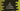# C# program to check if a number is armstrong or not## C# program to check if a number is armstrong or not:

In this C# program we will learn how to check if a number is armstrong or not. This program will take one number as input from the user, check if that is armstrong and print one message.

### What is Armstrong number:

A n digit number is called armstrong number of order n if the sum of powers of each digit to n of the number is equal to the number itself.

For example, 153 is a Armstrong number.

• It has three digits, so the value of n is 3.
• 1^3 + 5^3 + 3^3 = 1 + 125 + 27 = 153, i.e the number itself.

But, 154 is not a Armstrong number. Sum of powers of each digits of 154 to 3 is 1^3 + 5^3 + 4^3 = 1 + 125 + 64 = 190, which is not 154.

So, to find if a number is ArmStrong or not, we need to calculate:

• The total number of digits of the number and
• The sum of powers of each digit of the number.

Now, let’s write down the C# program to check for a ArmStrong number:

### C# program:

Below is the complete program:

``````using System;

public class Program
{
private static Boolean checkArmstrong(int num, int n){
int sum = 0;
int tempNum = num;
int rightDigit;

while(tempNum > 0){
rightDigit = tempNum % 10;
sum += Convert.ToInt32(Math.Pow(rightDigit, n));
tempNum /= 10;
}

return sum == num;
}

public static void Main()
{
int num;

Console.WriteLine("Enter a number:");

if(checkArmstrong(num, num.ToString().Length)){
Console.WriteLine("It is a Armstrong number");
}else{
Console.WriteLine("It is not a Armstrong number");
}
}
}``````

Here,

• num is an integer variable. We are asking the user to enter a number and this value is stored in this variable.
• checkArmstrong method is used to check if a number is armstrong or not. It takes two parameters. The first one is the number itself and the second one is the length of the number or total number of digits of the number.
• checkArmstrong returns one boolean value. Based on this value, we are printing one message mentioning this is armstrong or not.
• Inside checkArmstrong,

• We have created one sum variable to hold the sum of all powers. It is initialized as 0.
• Assigned the number to a different variable tempNumber.
• Using a while loop, we are finding the sum of all powers. Inside this loop, it picks the rightmost digit of the number, finds the power of this digit to n and adds it to sum.
• Once done, it returns one boolean value by comparing sum with num.

### Sample output:

If you run this program, it will print output as like below:

``````Enter a number:
407
It is a Armstrong number

Enter a number:
300
It is not a Armstrong number``````